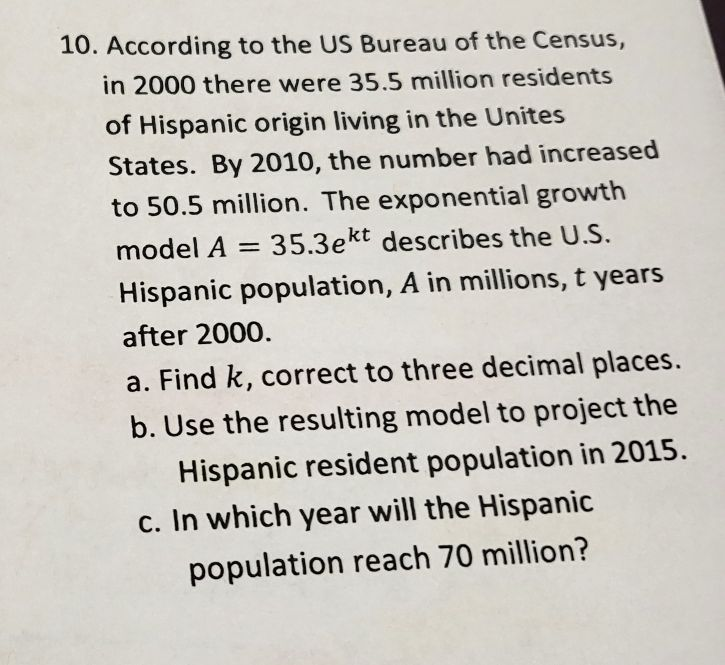### ¿Todavía tienes preguntas de matemáticas?

Pregunte a nuestros tutores expertos
Algebra
Pregunta0. According to the US Bureau of the Census, in $$2000$$ there were $$35.5$$ million residents of Hispanic origin living in the Unites States. By $$2010$$ , the number had increased to $$50.5$$ million. The exponential growth model $$A = 35.3 e ^ { k t }$$ describes the U.S. Hispanic population, A in millions, t years after $$2000$$ . a. Find $$k$$ , correct to three decimal places. b. Use the resulting model to project the Hispanic resident population in $$2015$$ . c. In which year will the Hispanic population reach $$70$$ million?